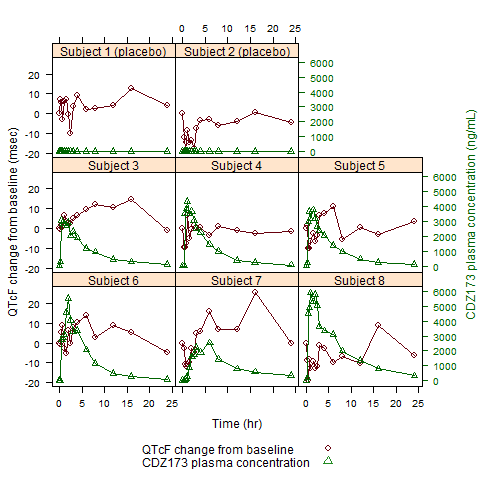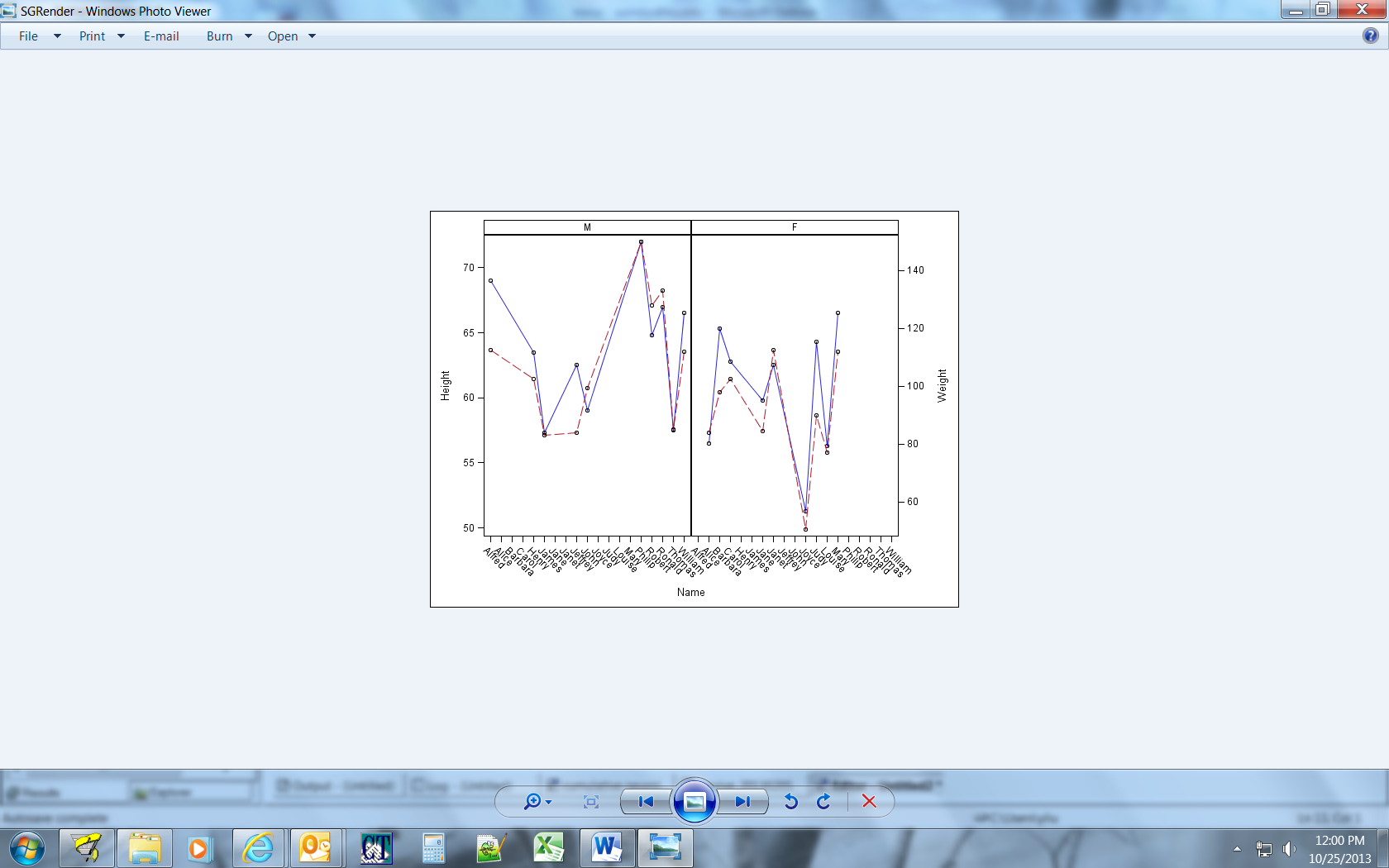## How could I get 2 y axis with 1 x axis for each plot in a panel?

Dear All,

I would like to plot the Drug PK concentration versus QTcF(a index of ECG) for each Subject over time. PK concentration will use the Y2 axis as the scale mark while QTcF use Y axis as the scale, the x axis will be used at the same time by PK and QTcF for each subject (see below graph), however I searched the Guidelines and found no way to do so. I guess the only option can reach this target is to use the GTL, but I am not experienced on this. I am wondering we need to use the Layout datapanel with Layout Overlay together in GTL?

Hope you can  help me.

Regards,

Frank1 ACCEPTED SOLUTION

Accepted SolutionsDanH_sas
SAS Super FREQ

## Re: How could I get 2 y axis with 1 x axis for each plot in a panel?

Here is a very simple example that you give you the ability to produce your plot. Let me know if you have any questions about it.

Thanks!

Dan

proc template;

define statgraph y2panel;

begingraph;

layout datapanel classvars=(sex) / columns=2 headerlabeldisplay=value;

layout prototype;

seriesplot x=name y=height / lineattrs=graphdata1 display=(markers);

seriesplot x=name y=weight / yaxis=y2 lineattrs=graphdata2 display=(markers);

endlayout;

endlayout;

endgraph;

end;

run;

proc sgrender data=sashelp.class template=y2panel; run;

10 REPLIES 10DanH_sas
SAS Super FREQ

## Re: How could I get 2 y axis with 1 x axis for each plot in a panel?

Here is a very simple example that you give you the ability to produce your plot. Let me know if you have any questions about it.

Thanks!

Dan

proc template;

define statgraph y2panel;

begingraph;

layout datapanel classvars=(sex) / columns=2 headerlabeldisplay=value;

layout prototype;

seriesplot x=name y=height / lineattrs=graphdata1 display=(markers);

seriesplot x=name y=weight / yaxis=y2 lineattrs=graphdata2 display=(markers);

endlayout;

endlayout;

endgraph;

end;

run;

proc sgrender data=sashelp.class template=y2panel; run;

## Re: How could I get 2 y axis with 1 x axis for each plot in a panel?

Hi Dan,

Below is waht I got by your code. why the graph on the left labeled as 'M'? isn't for all students? Thanks!DanH_sas
SAS Super FREQ

## Re: How could I get 2 y axis with 1 x axis for each plot in a panel?

Because some are male and some are female. To have only female names for the "F" cell and male names for the "M" cell, add COLUMNDATARANGE=UNION to the LAYOUT DATAPANEL after the "/". Sorry about the confusion.

-- DanDanH_sas
SAS Super FREQ

## Re: How could I get 2 y axis with 1 x axis for each plot in a panel?

Note, however, for the original use case that was posted, the COLUMNDATARANGE option should not be used. That option improves the output for the data I used in my example,

So cool!

Frank

## Re: How could I get 2 y axis with 1 x axis for each plot in a panel?

Dear Linlin,

I am very glad you can provide this question, which is helpful when I did my plot. Thank you.

Regards,

Frank

## Re: How could I get 2 y axis with 1 x axis for each plot in a panel?

Dear Dan,

I just read the SAS guideline book  and it impressed me only if we use the OVERYLAY layout, can we add the legend, is this the case? If so, does this there is no way to add the legend below the figure?

Thank you so much.

FrankDanH_sas
SAS Super FREQ

## Re: How could I get 2 y axis with 1 x axis for each plot in a panel?

Sorry, I forgot to add the legend. Here you go:

proc template;

define statgraph y2panel;

begingraph;

layout datapanel classvars=(sex) / columns=2 headerlabeldisplay=value;

layout prototype;

seriesplot x=name y=height / lineattrs=graphdata1 display=(markers) name="a";

seriesplot x=name y=weight / yaxis=y2 lineattrs=graphdata2 display=(markers) name="b";

endlayout;

sidebar / spacefill=false;

discretelegend "a" "b";

endsidebar;

endlayout;

endgraph;

end;

run;

proc sgrender data=sashelp.class template=y2panel; run;

## Re: How could I get 2 y axis with 1 x axis for each plot in a panel?

Dear Dan,

May I seek your answer to 'How can I re-value the scale in the y2 axis? For example, in the PROC GPLOT, we can set the values list in the axis property, but after I read the documentation, it seems we can not use this in PROC TEMPLATE'. Any comment are appreciated.

Regards,
FrankDanH_sas
SAS Super FREQ

## Re: How could I get 2 y axis with 1 x axis for each plot in a panel?

Yes, you can set the axis tick values in GTL. If you are in a DATA PANEL, like the example above, use the TICKVALUELIST option inside either the ROWAXISOPTS or COLUMNAXISOPTS option, depending on the axis you want to control. There is also a VIEWMIN/VIEWMAX option to control the limits of the axis. For layout OVERLAYs, you would use the same options inside of XAXISOPTS/YAXISOPTS/X2AXISOPTS/Y2AXISOPTS. Does this answer your question?

Thanks!

Dan

Discussion stats
• 10 replies
• 1406 views
• 5 likes
• 3 in conversation